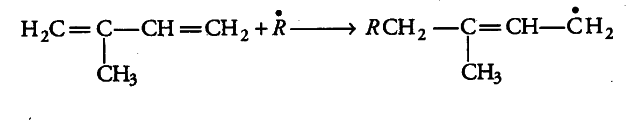# A monomer of a polymer upon ozonolysis gives one mole of methylglyoxal and two moles of formaldehyde

A monomer of a polymer upon ozonolysis gives one mole of methylglyoxal and two moles of formaldehyde.
(i) Identify the monomer of the polymer.
(ii) Give its free radical mode of addition polymerisation.

(i) As the monomer on ozonolysis gives one mole of methylglyoxal and two moles of formaldehyde, therefore, the monomer is isoprene.

(ii) The free radical mechanism of polymerisation of isoprene is given as follows:

(a) Chain initiation step Here, an initiator is used as free radical ® which initiate chain reaction as follows: